Courses

# Potential due to an Electric Dipole and Potential Due To A System Of Charges Class 12 Notes | EduRev

## Class 12 : Potential due to an Electric Dipole and Potential Due To A System Of Charges Class 12 Notes | EduRev

The document Potential due to an Electric Dipole and Potential Due To A System Of Charges Class 12 Notes | EduRev is a part of the Class 12 Course Physics Class 12.
All you need of Class 12 at this link: Class 12

Potential due to an Electric Dipole
The electric dipole is an arrangement which consists of two equal and opposite charges +q and -q separated by a small distance 2a.

Electric dipole moment is represented by a vector p of magnitude 2qa and this vector points in direction from -q to +q.

To find electric potential due to a dipole consider charge -q is placed at point P and charge +q is placed at point Q as shown below in the figure.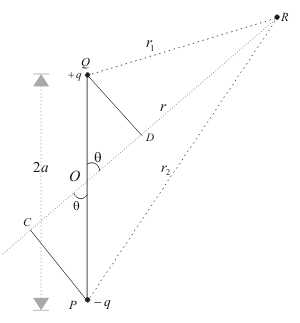Since electric potential obeys superposition principle so potential due to electric dipole as a whole would be sum of potential due to both the charges +q and -q. Thus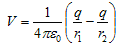where r1 and r2 respectively are distance of charge +q and -q from point R.
Now draw line PC perpandicular to RO and line QD perpandicular to RO as shown in figure. From triangle POC
cosθ=OC/OP = OC/a
therefore OC=acosθ similarly OD=acosθ
Now ,
r1 = QR≅RD = OR-OD = r-acosθ
r2 = PR≅RC = OR+OC = r+acosθ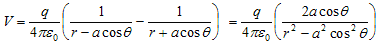since magnitude of dipole is

|p| = 2qa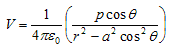If we consider the case where r>>a then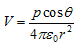again since pcosθ= p·rˆ where, rˆ is the unit vector along the vector OR then electric potential of dipole is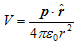for r>>a
From above equation we can see that potential due to electric dipole is inversly proportional to r2 not ad 1/r which is the case for potential due to single charge.
Potential due to electric dipole does not only depends on r but also depends on angle between position vector r and dipole moment p.

Potential Due To A System Of Charges
Consider a system of charges q1, q2,…, qn with position vectors r1, r2,…, r n relative to some origin. The potential V1 at P due to the charge q1 is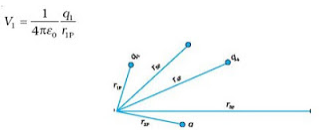where r1P is the distance between q and P. Similarly, the potential V2 at P due to q2 and due to q are given by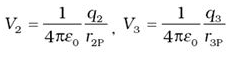where r2P and r3P are the distances of P from charges qand q3, respectively; and so on for the potential due to other charges.

By the superposition principle, the potential V at P due to the total charge configuration is the algebraic sum of the potentials due to the individual charges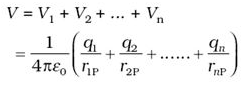The electric field outside the shell is as if the entire charge is concentrated at the centre. Thus, the potential outside the shell is given by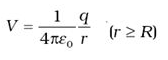where q is the total charge on the shell and R its radius. The electric field inside the shell is zero. This implies that potential is constant inside the shell (as no work is done in moving a charge inside the shell), and, therefore, equals its value at the surface, which is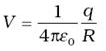Offer running on EduRev: Apply code STAYHOME200 to get INR 200 off on our premium plan EduRev Infinity!

,

,

,

,

,

,

,

,

,

,

,

,

,

,

,

,

,

,

,

,

,

;